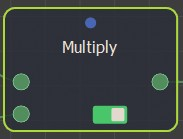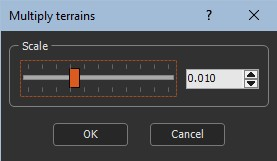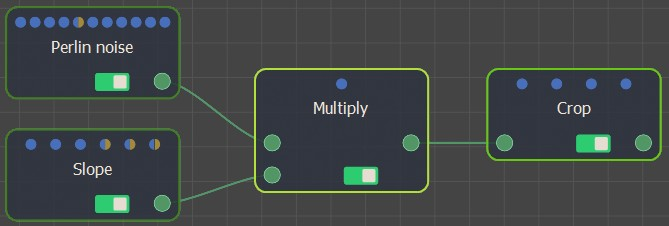### Adding a terrain Multiply node

This node multiplies two input terrains. At each vertex, the value of the output terrain is the multiplication of the values of both input terrains. This value, which can be extremely high, is then scaled down.

To add a node, right-click in the Graph Editor and select Create Node  > Terrain Composition > Multiply.Double click on the node to open its parameters:### Editing a terrain Multiply node

Edit the Multiply node in the parameters dialog:

• Scale: Sets the scale relative to zero.

### Linking to input terrains

Link the Multiply node to two input terrain nodes.If both input terrains do not have the same size or resolution, the size and resolution of the output terrain is determined by the size and resolution of the first terrain and the second terrain is enlarged or decreased so that its size corresponds exactly to the size of the output terrain, before being multiplied with the first terrain.

For example, the terrain from a Ridge noise node is multiplied with another Ridge noise (with a different seed) and outputs a terrain shown below.### Parameters

Parameter Use
Scale Scale factor to be applied

Copyright © 2021 · All Rights Reserved · Wysilab